and Jóhann Ísak

## Trig functions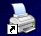Print

### Lesson 2      The Unit Circle

We begin by looking at a right angled triangle where the hypotenuse has a length of 1 unit.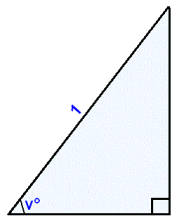Opposite side = sin v° Adjacent side  = cos v°

In a right angled triangle

sin v° = opposite side/hypotenuse

and

If the hypotenuse in a triangle has length 1 then it follows that

sin v° = opposite side and cos v° = adjacent side.

We now consider a circle drawn in a coordinate system.

A circle with a radius of 1 unit and it’s centre  in (0, 0) is called the Unit circle.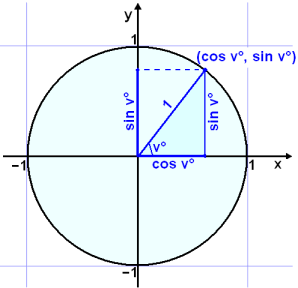The Unit circle

If we draw a radius that makes an angle of v° with the positive arm of the x axis and drop a perpendicular as the diagram shows we get a  right angled triangle with sides of length  cosv° and sin v°. This means that the coordinates of the point where the radius intersects the circle must be (cos v°, sin v°).

Now we’ll look at tan v° a similar way.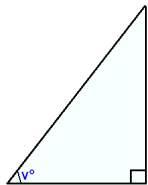opposite side = tan v° Adjacent side = 1

This time we choose the adjacent side to be of length 1 unit.

tan v° = opposite side/adjacent side.

The adjacent side = 1 therefore  tan v° = opposite side.

We can add this to the  diagram of the unit circle.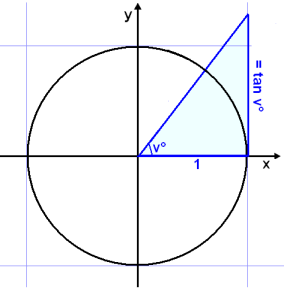To see the connection between sin, cos and tan we do the following calculations:

opposite side
tan v° =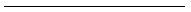opposite side/hypotenuse tan v° =adjacent side/hypotenuse Dividing the numerator and denominator by the hypotenuse.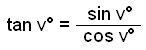The calculations we have shown so far apply to an angle drawn in the unit circle, measured from the x – axis and lying in the first quadrant of the coordinate system. If we call the point where the radius r cuts the circle P, and rotate the radius OP anticlockwise round the circle from the x axis we say that the angle v° is a positive rotation. If OP is rotated clockwise from the x axis we talk about a negative rotation. ( this is simply a definition that  has been agreed on). So if P moves along the circle up from the x axis we have a positive value for the rotation v. If P moves along the circle and down from the x axis we have a negative value for v.
( see the diagram).We now generalise the definition of sine, cosine and tangent as follows:

 If the radius OP in the unit circle is rotated  v° from the positive x-axis then      cos v° = x-coordinate of  P       sin v° = y-coordinate of  PThis definition implies that the trig functions can be positive or negative depending on in which quadrant of the coordinate system the point P lies.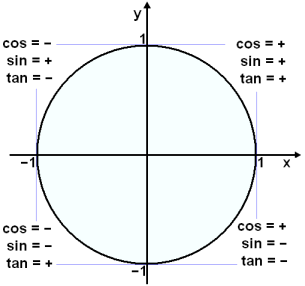As the point P moves with a positive rotation round the circle it reaches the same position every 360° . This means that the values of sine and cosine are repeated every 360°. Tangent  repeats it’s value every 180° as we will see in example 4.

### Example 1

Find the positive rotation that takes us to the same position as −200°

To do this we simply have to add on 360°.

−200° + 360° = 160°

### Example 2

Find an angle on the interval 0°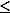v° < 360° equivalent to 1100°.

We need to subtract 360° several times until we reach the interval required.

1 circle:  1∙ 360° = 360°

2 circles:   2∙ 360° = 720°

3 circles:   3∙ 360° = 1080°

This is sufficient.

1100° − 1080° = 20°

### Example 3

We will now see how Pythagoras can be use to calculate some exact values for the trig functions.

First we look at 30°. The triangle in the diagram is half of an equilateral triangle and therefore we know that the opposite side is ½.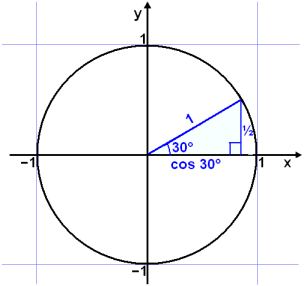sin 30° = ½ = 0,5 cos 2 30° + (½)2 = 12       cos 2 30°= 1 − ¼ = ¾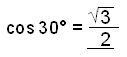≈ 0.866 Pythagoras rule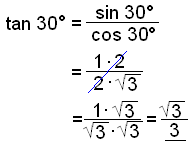0.577

Next we look at the angle 45°. The triangle is isosceles, we call the sides a.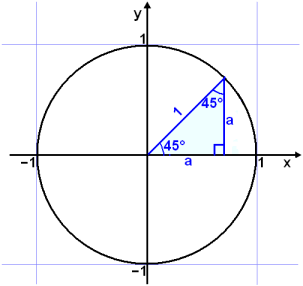a2 + a2 = 1 2a2 = 1 a2 = ½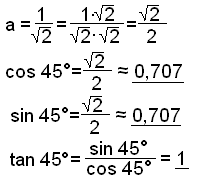Now the angle 60°.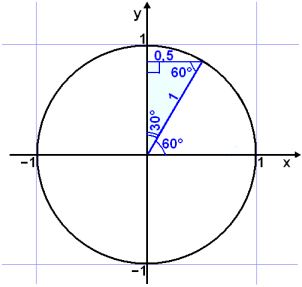Again we have a triangle with 30°, 60° and 90° so we can use the same calculations as for 30°. Notice that sine and cosine have exchanged values.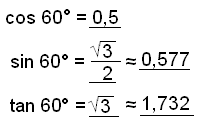The values of the trig functions for 90° can easily be seen from the  unit circle. OP, the arm of the angle lies on the y axis so the x-coordinate is 0 and the y coordinate is  1.

cos 90° = 0

sin 90° = 1

tan 90° = 1/0 does not exist.

Angles that are in other quadrants can be found by comparing them with angles in the first quadrant.120° lies in the second quadrant  (to the left of the positive y – axis) and therefore the cos 120° is negative, but the sin 120° is  positive..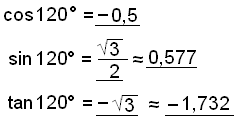We can continue in this way.

135° (90°+ 45°)  or ( 180°− 45°) can be found from 45°, only the signs change.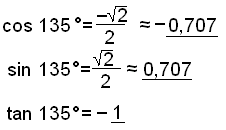### Example 4

Find the values of sine, cosine and tan for 225°.

225° is in the third quadrant and can be calculated from 45°. (45° + 180° = 225°). We begin by drawing a diagram.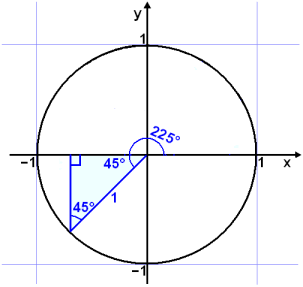We calculate in the same way as in example 3, both sine and cosine are negative but tan is positive (− divided by −).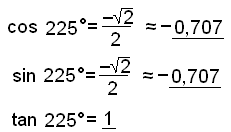Notice that tan 225°is the same as tan 45°. In other words the value of tan v° repeats itself every 180°.

### Example 5

Find all the solutions of the equation tan x° = 2, then write down which of these solutions are on the interval 0°x° < 360°.

Use the inverse tan function, tan −1, on your calculator.

tan −1(2) ≈ 63.44°

We know that the values of tan repeat themselves every 180°, so we can write a formula for all the solutions by adding k∙180° where k represents any whole number. The complete solution is therefore:

x° ≈ 63.44° + k∙180°          k is a whole number.

Two of these solutions lie  on the interval 0°x° < 360°. They are x° ≈ 63.44° and x° ≈ 63.44° + 180° ≈ 243.44°

### Example 6

Find the angles where sine takes the value 0.5 .

We first solve the equation sin v° = 0.5 using a calculator and the inverse sine function sin −1 . sin −1(0.5) = 30°. Now draw a diagram of the unit circle.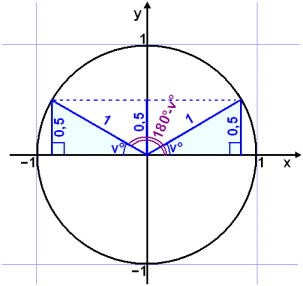Draw the angle v° as usual starting from the positive x axis and turning anticlockwise. The y coordinate of the point on the circle (height above the x axis ) is sin v°.
We are given this value 0.5 (sin v° = 0.5).

If we draw a horizontal line through this point (the dotted line in the diagram) we can see that there is a second point on the circle which has the same y coordinate and therefore the same value of sine. Both the shaded triangles in the diagram are congruent ( exactly the same). Measuring the rotation of this second radius from the positive x axis we get the second answer for v:

v°= 180° − 30° = 150°.

From this example we get the following rule:

 sin v° = sin (180°-v°)

We use this rule when we need to solve equations of the type

sin v° = a

Unfortunately calculators only give us one solution, the smallest angle that satisfies the equation measured from the positive x axis. We find the second answer by subtracting this answer from 180°. To find all possible solutions we need to add k∙360° to both solutions.
( k can be any whole number ).

### Example 7

Find all the solutions of the equation sin v° = −0.6.

Draw a diagram of the unit circle to see what we need to do.The calculator gives one solution sin −1(−0.6) ≈ −36.9°.

We get the second answer by subracting from 180°.

180° − (−36.9°) ≈ 216.9°

Add 360° to −36.9° to get rid of the negative.

−36.9° + 360° ≈ 323.1°

The complete soution is:   v1216.9° + k∙360° og v2323.1° + k∙360°

( It’s a good idea to use your calculators to check the answers.
sin 216.9° ≈ −0.6 and sin 323.1° ≈ −0.6 )

### Example 8

Solve the equation cos v° = 0.7.

Draw the unit circle to see what solutions there are.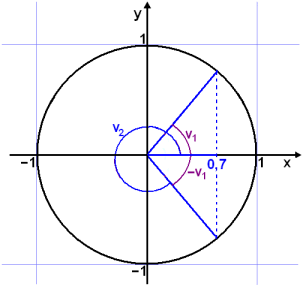In this example we are given the x coordinate ( cos v°= 0.7) of the point on the circle, that is the distance from the y axis. A perpendicular line ( dotted line) through the point where the radius intersects the circle has been drawn. This perpendicular line through 0.7 on the x axis cuts the circle in two points telling us there are two angles where cos v°= 0.7.

Using the calculator we get cos −1(0.7) ≈ 45.57°. We can see that the second angle is −45.57° . We find the  positive angle equivalent to this by adding 360°.

Solutions:  v145.57° + k∙360°

v2 ≈ −45.57° + 360° + k∙360° ≈ 314.43° + k∙360°

From this example we can see the following rule.

 cos v° = cos(-v°)

This is the rule we use to solve equations of the type

cos v° = a

### Example 9

Solve the inequality cos v° < −0.7 on the interval 0°v° < 360°.

First we solve the equation cos v° = −0.7.

The calculator gives cos −1(−0.7) ≈ 134.43° . The second solution is therefore −134.43°  or −134.43 + 360° ≈ 225.57°. Now we need to draw the unit circle.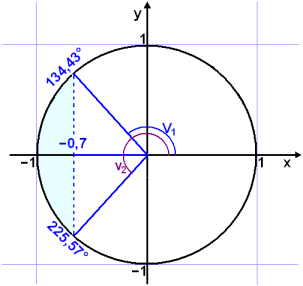We can see that cos v° is less than −0.7 if  v° is in the shaded area on the diagram.

The solution is therefore 134° < v°< 226°.

Try Quiz 2 on Trig functions.
Remember to use the checklist to keep track of your work.